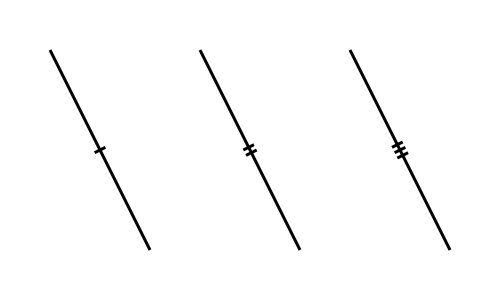# Tick

Martin McBride, 2022-01-18
Tags geometry tick
Categories generativepy generative artThe TickMarker class is a special Shape that adds a small cross line marker to a line, of the type often used in geometric diagrams to show that two lines are the same length. They look like this:You can add 1, 2 or 3 ticks.

The TickMarker only draws the tick, it doesn't draw the line itself. This would normally be draw using a Line object, Polygon object, or similar.

## TickMarker class

TickMarker is derived from the Shape class. It adds several methods:

• of_start_end
• with_length
• with_count
• with_gap

### of_start_end

Creates a marker based on a start and end points.

of_start_end(a, b)

Parameter Type Description
a (number, number) Tuple (x, y) for start of line
b (number, number) Tuple (x, y) for end of line

This will draw an tick marker for the line formed by ab. The tick will be halfway between points a and b.

### with_length

Sets the length of the tick.

with_length(length)

Parameter Type Description
length number Length of tick in user units.

The default is 4.

### with_count

Sets the number of ticks in the marker.

with_count(count)

Parameter Type Description
count int Number of ticks

This can be used to draw a single, double, or triple tick. Permitted values are 1, 2, or 3. Default is 1.

### with_gap

Sets the gap between the ticks if count > 1.

with_gap(gap)

Parameter Type Description
gap number Gap between ticks in user units.

Sets the spacing of the ticks. This is only relevant if there is more than one tick (ie if count > 1), otherwise it is ignored. The default is 1.

## tick function

This older tick function is deprecated as of GenerativePy 3.1.

tick(ctx, a, b, count=1, length=4, gap=1)

Parameter Type Description
ctx Context The Pycairo Context to draw to
a (number, number) Tuple (x, y) for point a
b (number, number) Tuple (x, y) for point b
count int Number of ticks on the line, 1, 2 or 3.
length number length of the tick in user units.
gap number Gap between ticks in user units.

Draws an tick across the line ab. The tick is half way between points a and b.

## Example

See the example in the angle_marker article.

If you found this article useful, you might be interested in the book Computer Graphics in Python or other books by the same author.

#### Popular tags

2d arrays abstract data type alignment and angle animation arc array arrays bar chart bar style behavioural pattern bezier curve built-in function callable object chain circle classes clipping close closure cmyk colour combinations comparison operator comprehension context context manager conversion count creational pattern data science data types decorator design pattern device space dictionary drawing duck typing efficiency ellipse else encryption enumerate fill filter font font style for loop function function composition function plot functools game development generativepy tutorial generator geometry gif global variable gradient greyscale higher order function hsl html image image processing imagesurface immutable object in operator index inner function input installing iter iterable iterator itertools join l system lambda function len lerp line line plot line style linear gradient linspace list list comprehension logical operator lru_cache magic method mandelbrot mandelbrot set map marker style matplotlib monad mutability named parameter numeric python numpy object open operator optimisation optional parameter or pandas partial application path pattern permutations pie chart polygon positional parameter print pure function python standard library radial gradient range recipes rectangle recursion reduce regular polygon repeat rgb rotation roundrect scaling scatter plot scipy sector segment sequence setup shape singleton slice slicing sound spirograph sprite square str stream string stroke structural pattern subpath symmetric encryption template text text metrics tinkerbell fractal transform translation transparency triangle truthy value tuple turtle unpacking user space vectorisation webserver website while loop zip